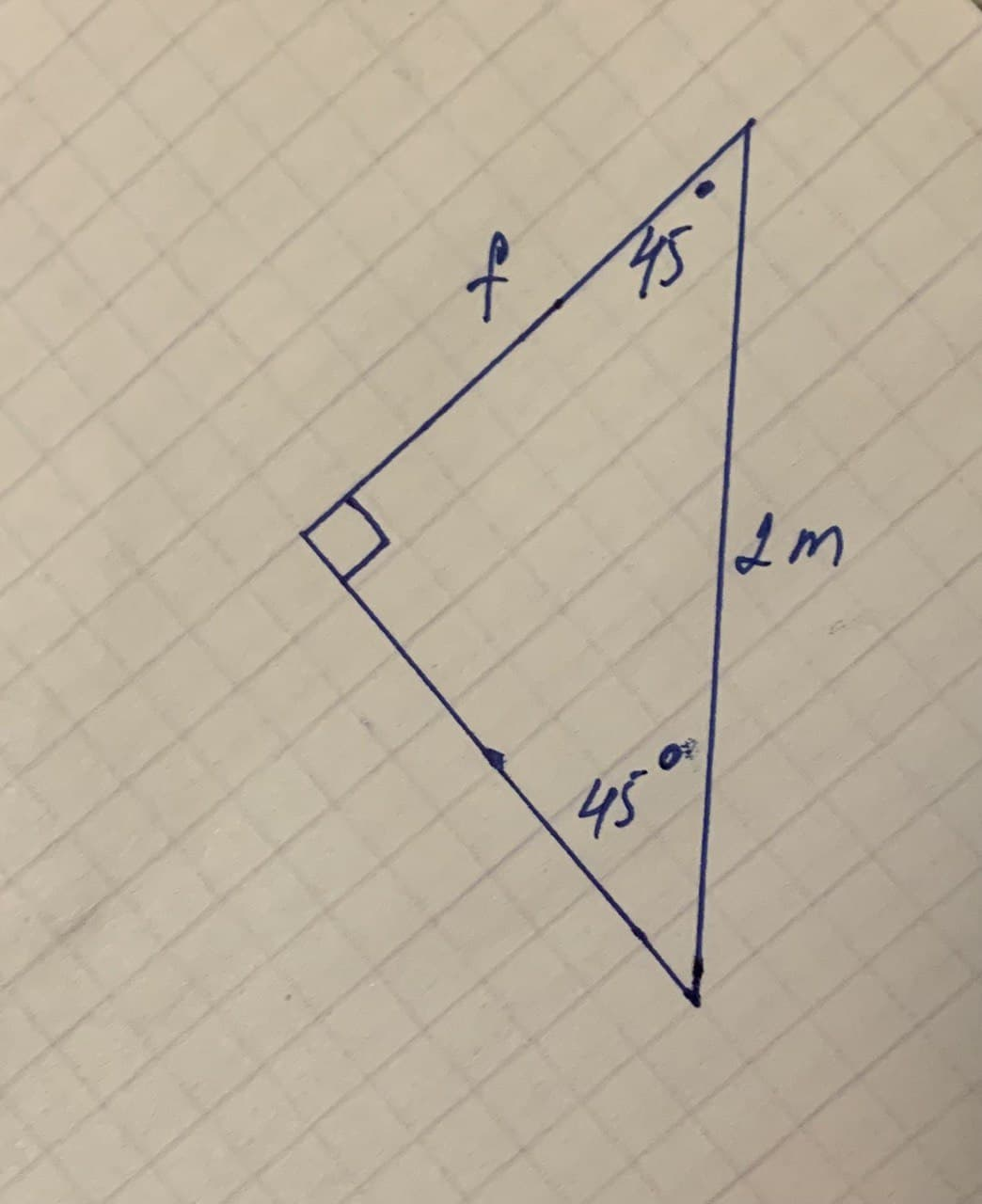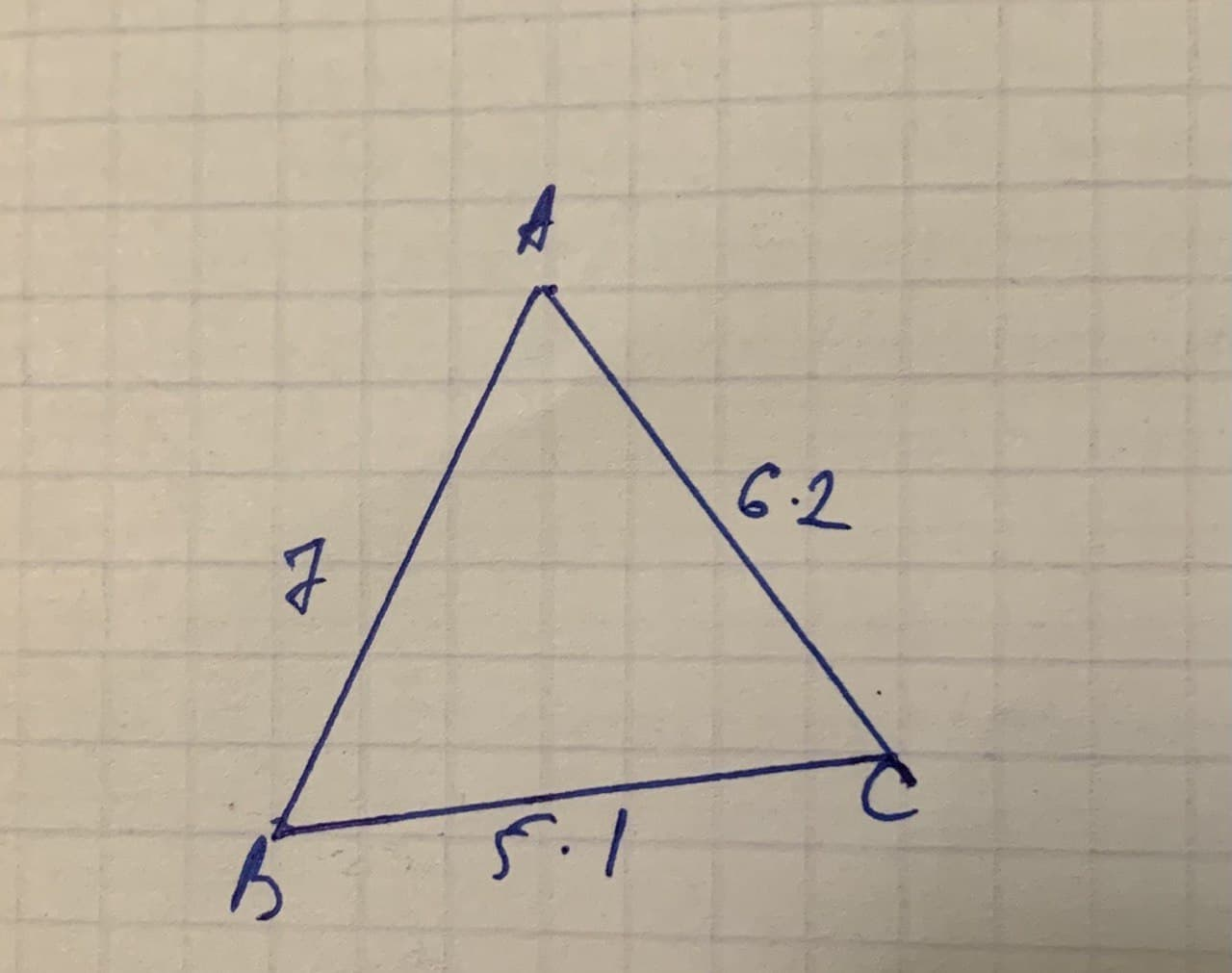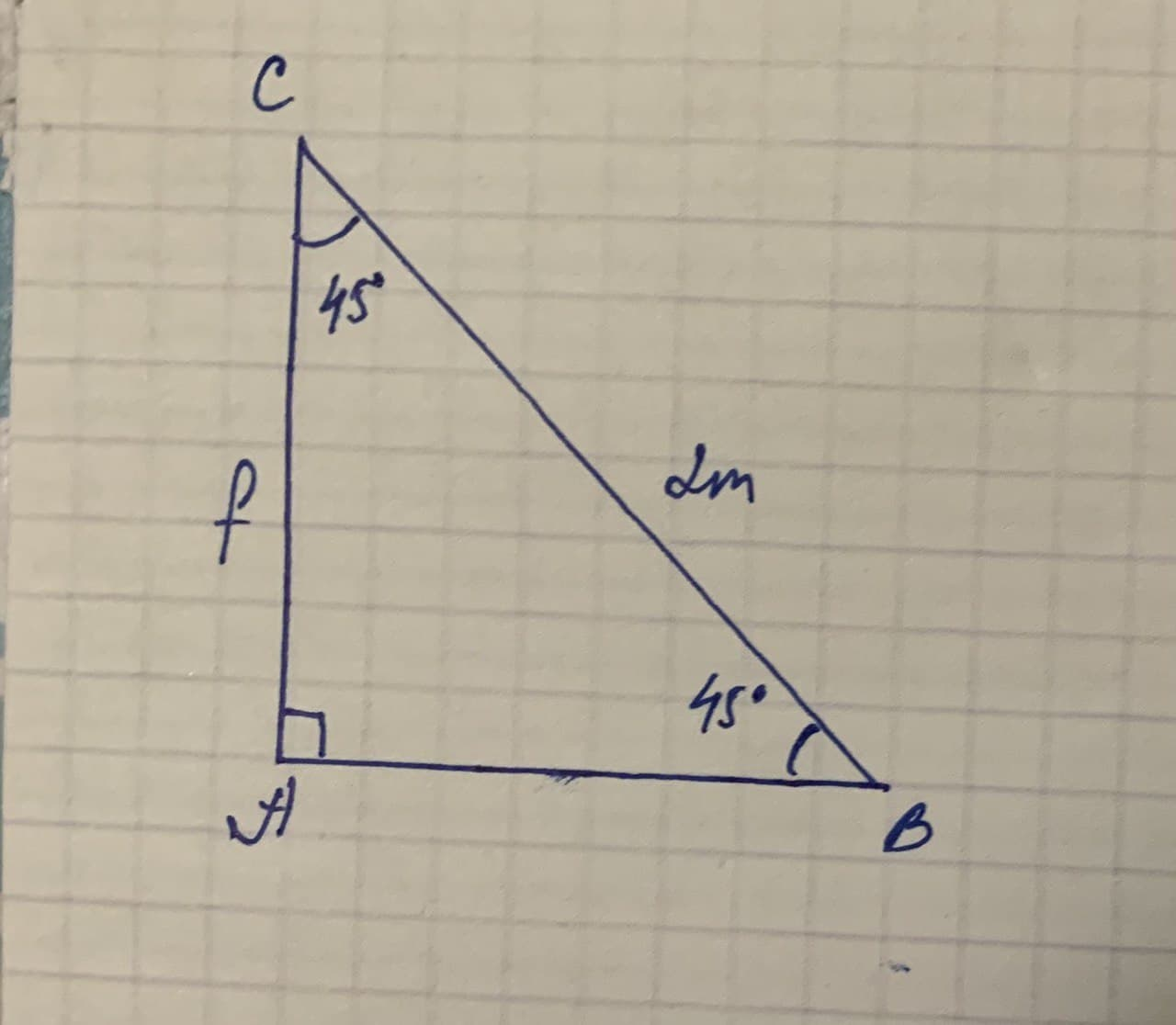The sides of triangle have lengths 7,5.1, and 6.2. WhatRena Giron 2021-11-22 Answered
The sides of triangle have lengths 7,5.1, and 6.2. What kind of triangle is it?
Find f.• Questions are typically answered in as fast as 30 minutes

Solve your problem for the price of one coffee

• Math expert for every subject
• Pay only if we can solve itRoger Noah
a) Given sides of triangle have lengths 7,5.1, and 6.2
Since all the sides are different.Let $$\displaystyle{c}={7}$$ (largest side)
$$\displaystyle{a}={5.1}$$ and $$\displaystyle{b}={6.2}$$
$$\displaystyle{c}^{{2}}={7}^{{2}}={49}{<}{\left({5.1}\right)}^{{2}}+{\left({6.2}\right)}^{{2}}={a}^{{2}}+{b}^{{2}}$$</span>
$$\displaystyle{c}^{{2}}{<}{a}^{{2}}+{b}^{{2}}$$</span>
if $$\displaystyle{c}^{{2}}{<}{a}^{{2}}+{b}^{{2}}$$</span>, then the triangle is an acute triangle
Acute b) Since $$\displaystyle{m}\angle{A}{C}{B}={m}\angle{A}{B}{C}={4}{s}$$$$\displaystyle\Rightarrow{A}{B}={A}{C}={f}$$
$$\displaystyle{A}{B}={f}$$
$$\displaystyle{9}{n}\triangle{A}{B}{C},{A}{C}^{{2}}={A}{B}^{{2}}+{A}{C}^{{2}}$$
$$\displaystyle={f}^{{2}}+{f}^{{2}}={2}{f}^{{2}}$$
$$\displaystyle\Rightarrow{4}={2}{f}^{{2}}\Rightarrow{f}^{{2}}={2}$$
$$\displaystyle{f}=\sqrt{{{2}}}={1.414}$$
$$\displaystyle{f}={1.414}$$